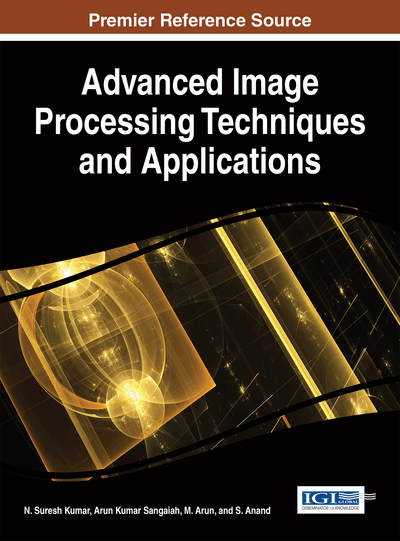# A Technique to Approximate Digital Planar Curve with Polygon

Mangayarkarasi Ramaiah (VIT University, India) and Bimal Kumar Ray (VIT University, India)
DOI: 10.4018/978-1-5225-2053-5.ch007
Available
\$37.50
No Current Special Offers

## Abstract

This chapter presents a technique which uses the sum of height square as a measure to define the deflection associated with a pseudo high curvature points on the digital planar curve. The proposed technique iteratively removes the pseudo high curvature points whose deflection is minimal, and recalculates the deflection associated with its neighbouring pseudo high curvature points. The experimental results of the proposed technique are compared with recent state of the art iterative point elimination methods. The comparative results show that the proposed technique produces the output polygon in a better way than others for most of the input digital curve.
Chapter Preview
Top

## Introduction

There had been significant interest in polygonal approximation of digital planar curve in the last two decades and it resulted in a large number of published papers. Another reason for its popularity is its application to a large number of problems viz. identifying registration number on cars (2002), for portrayal of geographical information (1998), recognizing handwritten forms (2016), in handling biological signal (electroocluography) (1990), in exploration of image (2002) and in matching similar images (1994, 1987, 1993).The technique polygonal approximation is classified as sequential, split and merge and heuristic search. The sequential algorithms use a linear probe to calculate error condition and if the condition is found to be false then the search for a new segment is started. Skalansky and Gonzalez (1980) and Ray and Ray (1994) presented sequential technique that performs a single pass along the digital curve and finds the vertices (pseudo high curvature vertices) of the closed curve. Skalansky and Gonzalez (1980) use perpendicular distance as a measure to determine the vertices whereas Ray and Ray (1994), in an attempt to determine the longest promising line segment along a digital planar curve with minimum possible error optimize an objective function involving sum of squares of error and length of line segment. The output curve produced by Skalansky and Gonzalez (1980) depends upon the starting vertex of the input curve. Sequential techniques may not be able to retain features such as sharp corners and spikes. In contrast to sequential techniques, recursive splitting technique is based on divide-and-conquer method.

Pavlidis and Horowitz (1974) use split and merge technique which fits a line to an initial segmentation of the boundary vertices and computes the least squares error. It iteratively splits a curve if the error is too large and merges two segments if the error is too small. Dunham (1986) suggests an optimal algorithm (using dynamic programming), which instead of specifying the number of line segments specify the error and determine the minimum number of line segments. The recurrence relation used to determine the minimum number of line segments is simple. Sato (1992) also used dynamic programming to find the optimal approximating closed curve.

Yin (1998) presents a method for polygonal approximation that uses genetic algorithm. Yin (2003) presents Tabu search technique to reduce time and space complexity in polygonal approximation, but it is found to be computationally expensive.

The technique proposed in this chapter doesn’t fall into anyone of the categories described above. The main objective of this chapter is to introduce a new measure to define the deflection associated with pseudo high curvature vertex more precisely than the other recent iterative vertex elimination techniques. The technique proposed in this chapter obtains the pseudo high curvature vertex using chain code and deletes the duplicate vertices on the digital curve iteratively and produce output polygon retaining with real vertices.

## Complete Chapter List

Search this Book:
Reset